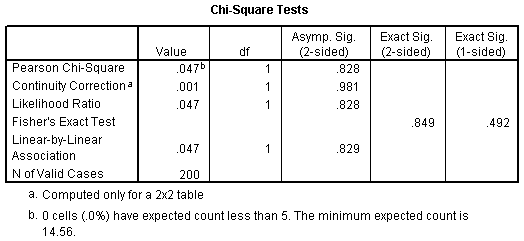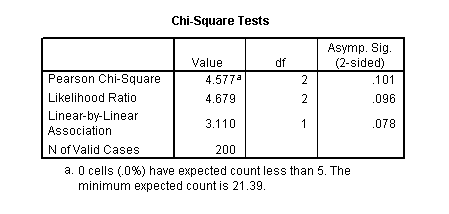## Chi-square test

A chi-square test is used when you want to see if there is a relationship between two categorical variables. In SPSS, the chisq option is used on the statistics subcommand of the crosstabs command to obtain the test statistic and its associated p-value. Using the hsb2 data file, let’s see if there is a relationship between the type of school attended (schtyp) and students’ gender (female). Remember that the chi-square test assumes that the expected value for each cell is five or higher. This assumption is easily met in the examples below. However, if this assumption is not met in your data, please see the section on Fisher’s exact test below.

crosstabs /tables = schtyp by female /statistic = chisq.Let’s look at another example, this time looking at the linear relationship between gender (female) and socio-economic status (ses). The point of this example is that one (or both) variables may have more than two levels, and that the variables do not have to have the same number of levels. In this example, female has two levels (male and female) and ses has three levels (low, medium and high).

crosstabs /tables = female by ses /statistic = chisq.

These results indicate that there is no statistically significant relationship between the type of school attended and gender (chi-square with one degree of freedom = 0.047, p = 0.828).Again we find that there is no statistically significant relationship between the variables (chi-square with two degrees of freedom = 4.577, p = 0.101).